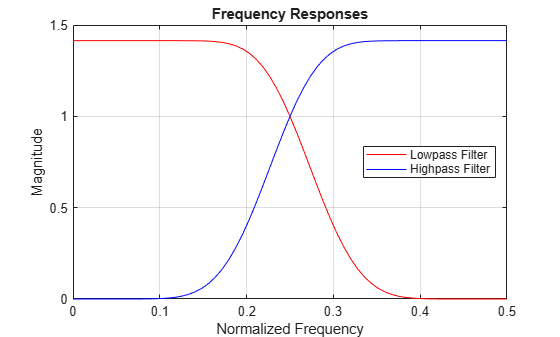# qmf

Scaling and wavelet filter

## Syntax

``Y = qmf(X)``
``Y = qmf(X,P)``

## Description

````Y = qmf(X)` changes the signs of the even-indexed elements of the reversed vector filter coefficients `X`.```

example

````Y = qmf(X,P)` changes the signs of the even-indexed elements of the reversed vector filter coefficients `X` if `P` is `0`. If `P` is `1`, the signs of the odd-indexed elements are reversed. Changing `P` changes the phase of the Fourier transform of the resulting wavelet filter by π radians.```

## Examples

collapse all

This example shows how to create a quadrature mirror filter associated with the `db10` wavelet.

Obtain the scaling filter associated with the `db10` wavelet.

`sF = dbwavf("db10");`

`dbwavf` normalizes the filter coefficients so that the norm is equal to $1/\sqrt{2}$. Normalize the coefficients so that the filter has norm equal to 1.

`G = sqrt(2)*sF;`

Obtain the wavelet filter coefficients by using `qmf`. Plot the filters.

```H = qmf(G); subplot(2,1,1) stem(G) title("Scaling (Lowpass) Filter G") grid on subplot(2,1,2) stem(H) title("Wavelet (Highpass) Filter H") grid on```Save the current extension mode. Set the extension mode to Periodization. Generate a random signal of length 64. Perform a single-level wavelet decomposition of the signal using `G` and `H`. For purposes of reproducibility, set the random seed to the default value.

```origmode = dwtmode("status","nodisplay"); dwtmode("per","nodisplay") n = 64; rng default sig = randn(1,n); [a,d] = dwt(sig,G,H);```

The lengths of the approximation and detail coefficients are both 32. Confirm that the filters preserve energy.

`[sum(sig.^2) sum(a.^2)+sum(d.^2)]`
```ans = 1×2 92.6872 92.6872 ```

Compute the frequency responses of `G` and `H`. Zeropad the filters when taking the Fourier transform.

```n = 128; F = 0:1/n:1-1/n; Gdft = fft(G,n); Hdft = fft(H,n);```

Plot the magnitude of each frequency response.

```figure plot(F(1:n/2+1),abs(Gdft(1:n/2+1)),"r") hold on plot(F(1:n/2+1),abs(Hdft(1:n/2+1)),"b") grid on title("Frequency Responses") xlabel("Normalized Frequency") ylabel("Magnitude") legend("Lowpass Filter","Highpass Filter","Location","east") hold off```Confirm the sum of the squared magnitudes of the frequency responses of `G` and `H` at each frequency is equal to 2.

```sumMagnitudes = abs(Gdft).^2+abs(Hdft).^2; [min(sumMagnitudes) max(sumMagnitudes)]```
```ans = 1×2 2.0000 2.0000 ```

Confirm that the filters are orthonormal.

```df = [G;H]; id = df*df'```
```id = 2×2 1.0000 -0.0000 -0.0000 1.0000 ```

Restore the original extension mode.

`dwtmode(origmode,"nodisplay")`

This example shows the effect of setting the phase parameter of the `qmf` function.

Obtain the decomposition lowpass filter associated with a Daubechies wavelet.

`lowfilt = wfilters("db4");`

Use the `qmf` function to obtain the decomposition lowpass filter for a wavelet. Then, compare the signs of the values when the `qmf` phase parameter is set to 0 or 1. The reversed signs indicates a phase shift of $\pi$ radians, which is the same as multiplying the DFT by ${e}^{i\pi }$.

`p0 = qmf(lowfilt,0)`
```p0 = 1×8 0.2304 -0.7148 0.6309 0.0280 -0.1870 -0.0308 0.0329 0.0106 ```
`p1 = qmf(lowfilt,1)`
```p1 = 1×8 -0.2304 0.7148 -0.6309 -0.0280 0.1870 0.0308 -0.0329 -0.0106 ```

Compute the magnitudes and display the difference between them. Unlike the phase, the magnitude is not affected by the sign reversals.

`abs(p0)-abs(p1)`
```ans = 1×8 0 0 0 0 0 0 0 0 ```

## Input Arguments

collapse all

Filter coefficients, specified as a vector.

Data Types: `single` | `double`

Phase parameter, specified as follows.

• `0` — Change signs of even-indexed elements of the reversed vector `X`

• `1` — Change signs of odd-indexed elements of the reversed vector `X`

Data Types: `single` | `double`

collapse all

Let `x` be a finite energy signal. Two filters F0 and F1 are quadrature mirror filters (QMF) if, for any x,

`${‖{y}_{0}‖}^{2}+{‖{y}_{1}‖}^{2}={‖x‖}^{2}$`

where y0 is a decimated version of the signal x filtered with F0, so y0 is defined by x0 = F0(x) and y0(n) = x0(2n). Similarly, y1 is defined by x1 = F1(x) and y1(n) = x1(2n). This property ensures a perfect reconstruction of the associated two-channel filter banks scheme (see  p. 103).

For example, if F0 is a Daubechies scaling filter with norm equal to 1 and F1 = `qmf`(F0), then the transfer functions F0(z) and F1(z) of the filters F0 and F1 satisfy the condition:

`$|{F}_{0}\left(z\right){|}^{2}+|{F}_{1}\left(z\right){|}^{2}=2.$`

 Strang, Gilbert, and Truong Nguyen. Wavelets and Filter Banks. Rev. ed. Wellesley, Mass: Wellesley-Cambridge Press, 1997.

## Support#### Try MATLAB, Simulink, and Other Products

Get trial now